Note: The other languages of the website are Google-translated. Back to English

## 计算列中的唯一数值或日期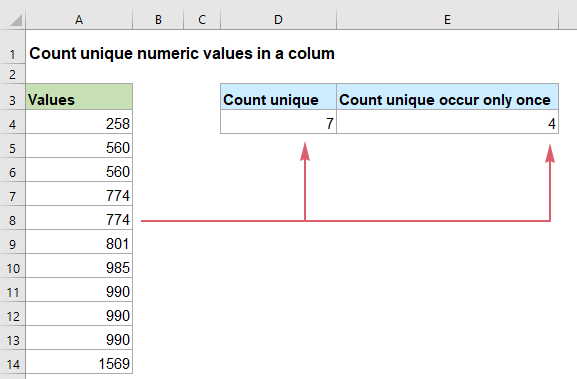#### 在 Excel 2019、2016 及更早版本中计算列中的唯一数值或日期

##### 计算列中的唯一数值或日期

=SUM(--(FREQUENCY(range,range)>0))
• range：要从中计算唯一值的数据列。

=SUM(--(FREQUENCY(A2:A12,A2:A12)>0))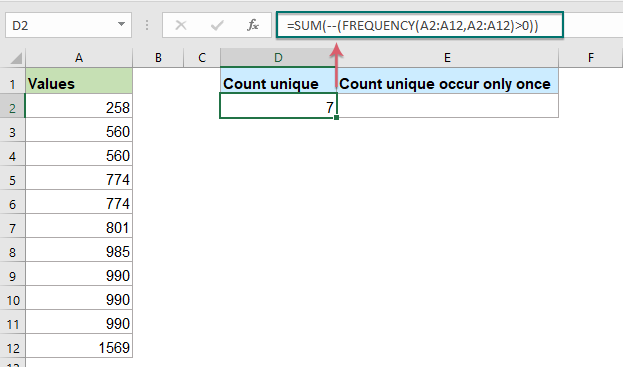##### 公式说明：

=SUM(--(FREQUENCY(A2:A12,A2:A12)>0))

• 频率(A2:A12,A2:A12)：FREQUENCY 函数返回列表 A2:A12 中每个数值的计数，它会得到一个这样的数组：{1;2;0;2;0;1;1;3;0;0;1; 0}。 数组中的数字表示值在列中出现的次数。 而数组中的数字 0 表示该数字已经出现在数据范围内。
• --(FREQUENCY(A2:A12,A2:A12)>0=--({1;2;0;2;0;1;1;3;0;0;1;0})>0：数组中的每个值都将与零进行比较：如果大于 0，则显示 TRUE，否则显示 FALSE。 然后，双负号将 TRUE 和 FALSE 转换为 1 和 0，因此，它将返回结果为：{1;1;0;1;0;1;1;1;0;0;1; 0}。
• SUM(--(FREQUENCY(A2:A12,A2:A12)>0))=SUM({1;1;0;1;0;1;1;1;0;0;1;0}): 最后，SUM 函数将数组中的所有值相加，得到结果：7。

##### 计算在列中仅出现一次的唯一数值或日期

{=SUM(IF(ISNUMBER(range)*COUNTIF(range,range)=1,1,0))}
Array formula, should press Ctrl + Shift + Enter keys together.
• range：要从中计算唯一值的数据列。

=SUM(IF(ISNUMBER(A2:A12)*COUNTIF(A2:A12,A2:A12)=1,1,0))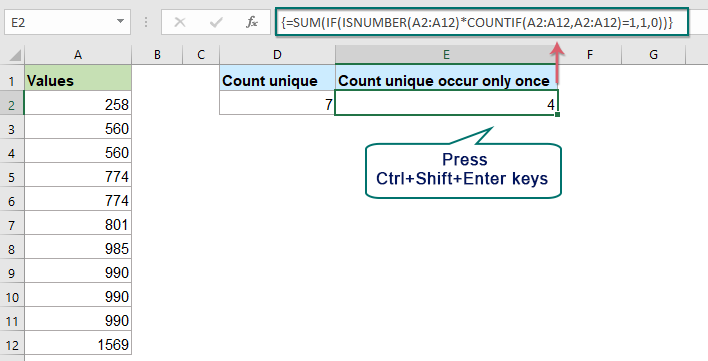##### 公式说明：

=SUM(IF(ISNUMBER(A2:A12)*COUNTIF(A2:A12,A2:A12)=1,1,0))

• ISNUMBER(A2:A12)：此ISNUMBER 函数用于检查列表A2:A12 中的值是否为数字。 如果单元格包含数值，则返回 TRUE，否则返回 FALSE。 所以，你会得到一个这样的数组：{TRUE;TRUE;TRUE;TRUE;TRUE;TRUE;TRUE;TRUE;TRUE;TRUE;TRUE}。
• 计数(A2:A12,A2:A12): COUNTIF 函数计算每个值在特定范围内出现的次数，它会返回这样的结果：{1;2;2;2;2;1;1;3;3;3;1}。
• ISNUMBER(A2:A12)*COUNTIF(A2:A12,A2:A12)= {TRUE;TRUE;TRUE;TRUE;TRUE;TRUE;TRUE;TRUE;TRUE;TRUE;TRUE}* {1;2;2;2;2;1;1;3;3;3;1}：这两个表达式相乘得到这个结果：{1;2;2;2;2;1;1;3;3;3;1}。
• IF(ISNUMBER(A2:A12)*COUNTIF(A2:A12,A2:A12)=1,1,0)=IF({1;2;2;2;2;1;1;3;3;3;1}=1,1,0): 这里 IF 函数检查数组中的每个值是否等于 1：如果是，则返回数字 1； 如果不是，则显示数字 0。 结果是这样的：{1;0;0;0;0;1;1;0;0;0;1}。
• SUM(IF(ISNUMBER(A2:A12)*COUNTIF(A2:A12,A2:A12)=1,1,0))=SUM({1;0;0;0;0;1;1;0;0;0;1})：最后，SUM 函数将数组中的值相加，并返回唯一值的总数：4。

#### 在 Excel 365 中计算列中的唯一数值或日期

##### 计算列中的唯一数值或日期

=COUNT(UNIQUE(range))
• range：要从中计算唯一值的数据列。

=COUNT(UNIQUE(A2:A12))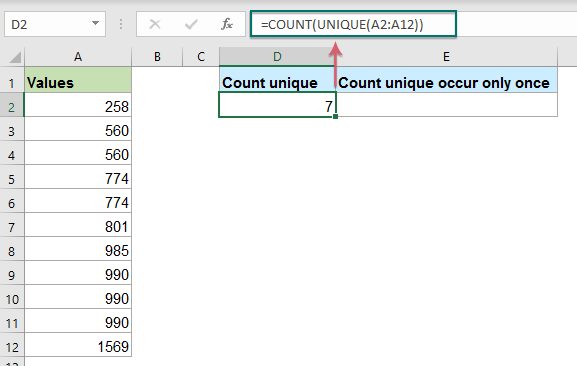##### 公式说明：

=计数（唯一（A2：A12））

• 独一无二(A2:A12)：此 UNIQUE 函数从列表中提取所有唯一值，您将获得一个数组列表，如下所示：{258;560;774;801;985;990;1569}。
• COUNT(UNIQUE(A2:A12))=COUNT({258;560;774;801;985;990;1569})：这个 COUNT 函数计算数值的个数并返回结果：7。

##### 计算在列中仅出现一次的唯一数值或日期

=COUNT(UNIQUE(range,0,1))
• range：要从中计算唯一值的数据列。

=COUNT(UNIQUE(A2:A12,0,1))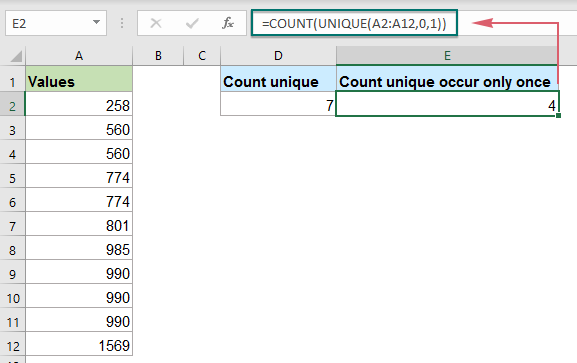##### 公式说明：

=计数（唯一（A2：A12,0,1））

• 独一无二(A2:A12,0,1)：此 UNIQUE 函数从列表中提取仅出现一次的唯一值，您将获得一个数组列表，如下所示：{258;801;985;1569}。
• COUNT(UNIQUE(A2:A12,0,1))=COUNT({258;801;985;1569})：这个 COUNT 函数计算数值的个数并返回结果：4。

#### 使用的相对功能：

• 和：
• Excel SUM 函数返回提供的值的总和。
• 频率：
• FREQUENCY 函数计算值在一个值范围内出现的频率，然后返回一个垂直的数字数组。
• 编号：
• Excel ISNUMBER 函数在单元格包含数字时返回 TRUE，否则返回 FALSE。
• 计数：
• COUNTIF函数是Excel中的统计函数，用于计算符合条件的单元格数。
• 数数：
• COUNT 函数用于计算包含数字的单元格的数量，或计算参数列表中的数字。
• 独特的：
• UNIQUE 函数返回列表或范围中的唯一值列表。

#### 更多文章：

• 根据条件计算唯一数值
• 在 Excel 工作表中，您可能会遇到根据特定条件计算唯一数值数量的问题。 例如，如何从报告中计算产品“T 恤”的唯一数量值，如下图所示？ 在本文中，我将展示一些在 Excel 中实现此任务的公式。
• 计数单元格数等于多个值之一
• 假设，我在 A 列中有一个产品列表，现在，我想从 A 列中获取列在 C4:C6 范围内的特定产品 Apple、Grape 和 Lemon 的总数，如下图所示。 通常，在 Excel 中，简单的 COUNTIF 和 COUNTIFS 函数在这种情况下不起作用。 这篇文章，我将谈谈如何通过 SUMPRODUCT 和 COUNTIF 函数的组合快速轻松地解决这个工作。
• 计算包含特定字符数的单元格数
• 如果您想计算在一系列单元格中包含一定数量字符（例如 5 个字符）的单元格数量，如下图所示。 在 Excel 中，COUNTIF 函数可以帮到您。 在本文中，我将讨论如何获取恰好包含 x 个字符的单元格或包含超过一定数量字符的单元格的数量。

### 最佳办公效率工具

#### Kutools for Excel-帮助您从人群中脱颖而出

• 专为1500个工作方案而设计，可帮助您解决80％的Excel问题。
• 每天减少成千上万的键盘和鼠标点击，减轻您疲倦的眼睛和手部的疲劳。
• 在3分钟内成为一名Excel专家。 不再需要记住任何痛苦的公式和VBA代码。
• 30天无限制免费试用。 60天退款保证。 免费升级和支持2年。#### Office选项卡-在Microsoft Office（包括Excel）中启用选项卡式阅读和编辑

• 一秒钟即可在数十个打开的文档之间切换！
• 每天为您减少数百次鼠标单击，告别鼠标手。
• 查看和编辑多个文档时，可将您的工作效率提高50％。
• 像Chrome，Firefox和新的Internet Explorer一样，为Office（包括Excel）带来高效选项卡。0  产品特性